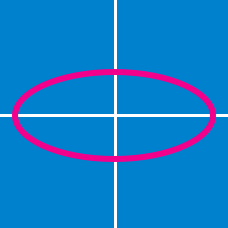Geometry

# Conic Sections: Level 5 Challenges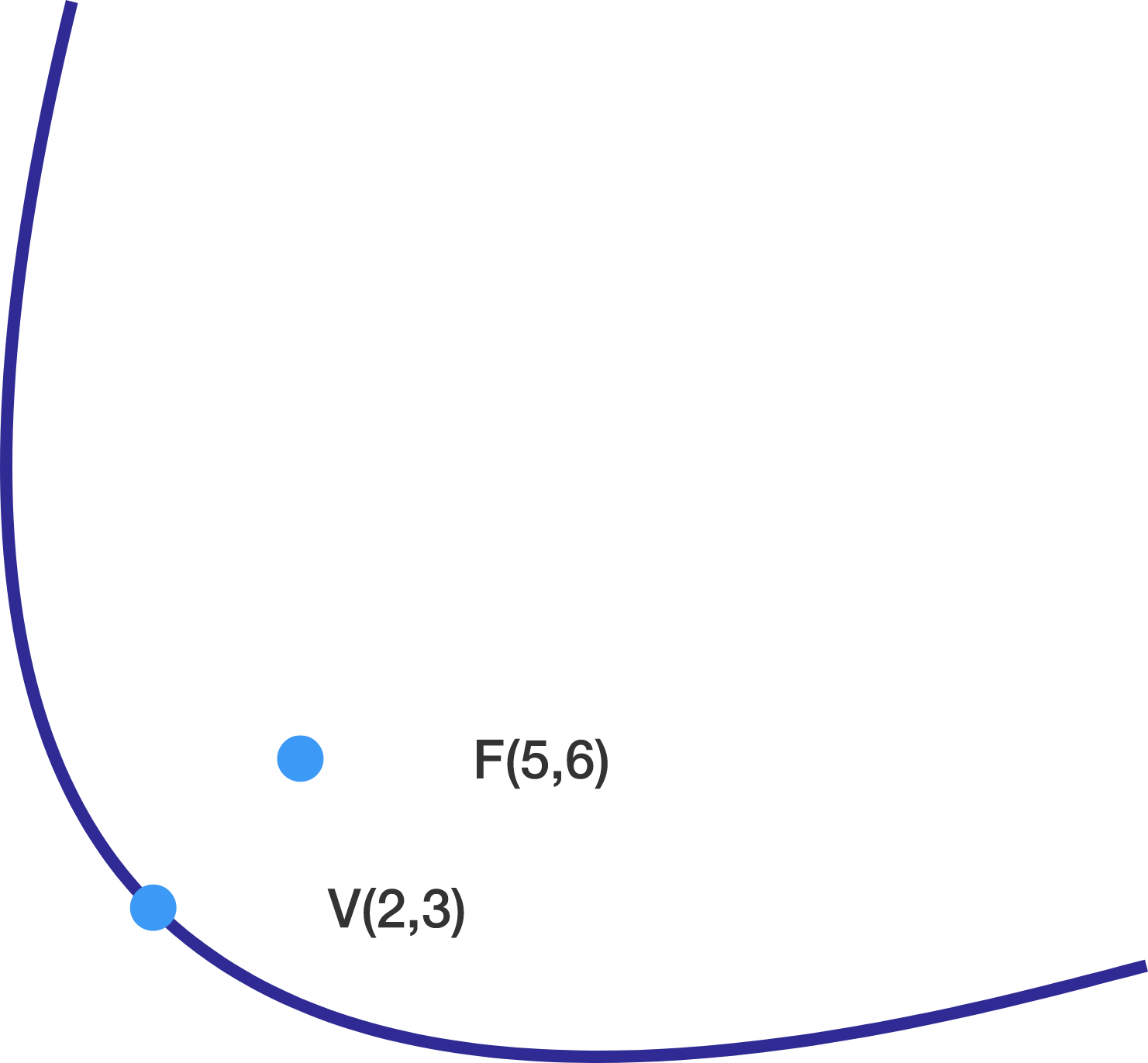A parabola whose vertex is the point $V=(2,3)$ and whose focus is $(5,6)$ has equation $ax^2+bxy+cy^2+dx+ey+f=0$, where $\gcd(a,b,c,d,e,f)=1$.

Find $\big|a+b+c+d+e+f\big|.$

Given the ellipse $3x^{2} - 12x + 2y^{2} + 12y + 6 = 0$, there exists a real number $k = a\sqrt{b} + c$, (where $a,b,c$ are all positive integers and $b$ is square-free), such that the hyperbola $xy - 2y + 3x = k$ is tangent to the ellipse at two points.

Find $a + b + c$.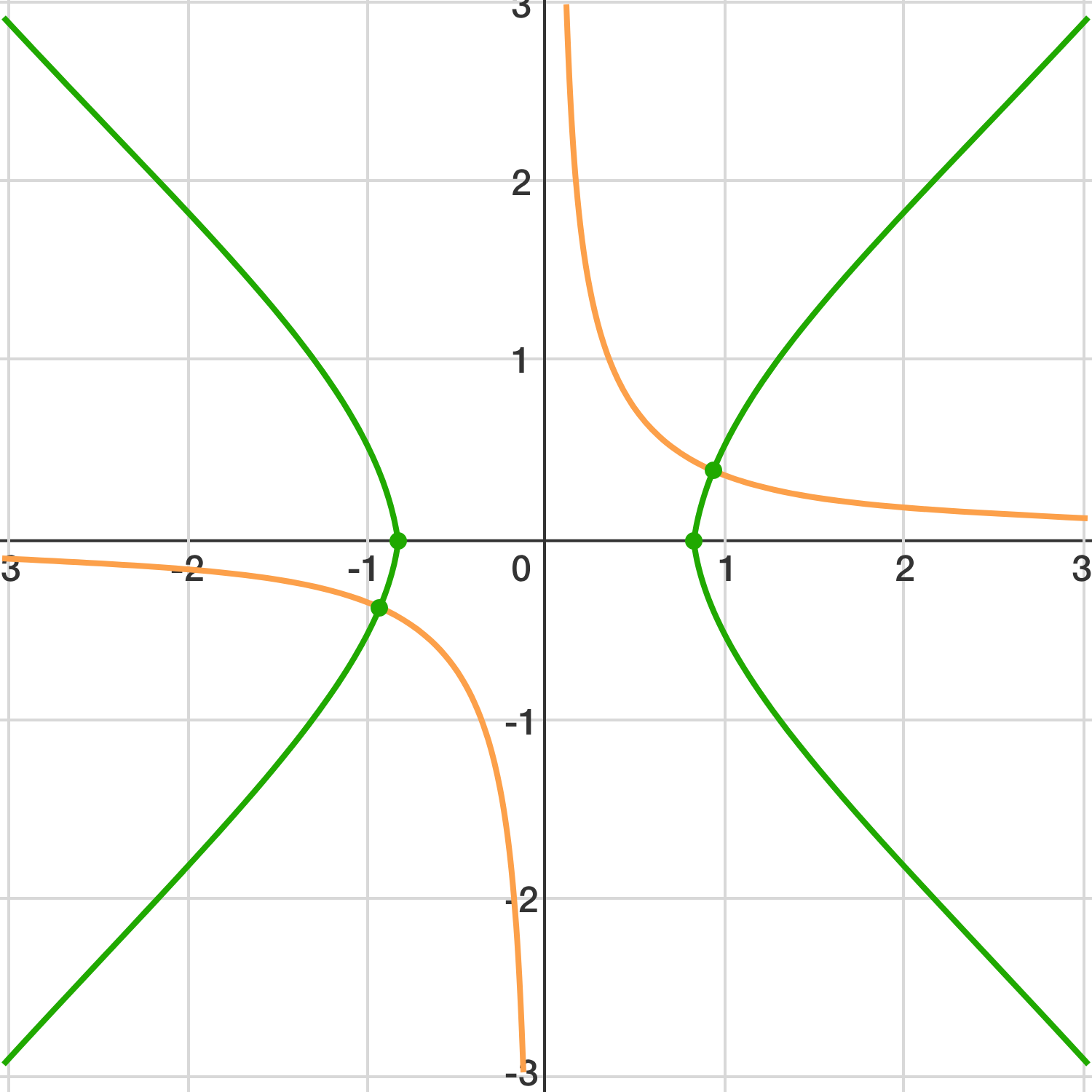A hyperbola $H$ with equation $xy=n$ (where $n\le 1000$) is rotated $45^{\circ}$ to obtain the hyperbola $H'$. Let the positive difference between the number of lattice points on $H$ and $H'$ be $D$. Given that both $H$ and $H'$ have at least one lattice point, find the maximum possible value of $D$.

Details and Asumptions: A lattice point is a point that has integer $x$- and $y$-coordinates.

You may want to look at the list of Highly Composite Numbers.

A circle and an ellipse of the same area share the interior of a larger circle, without overlap.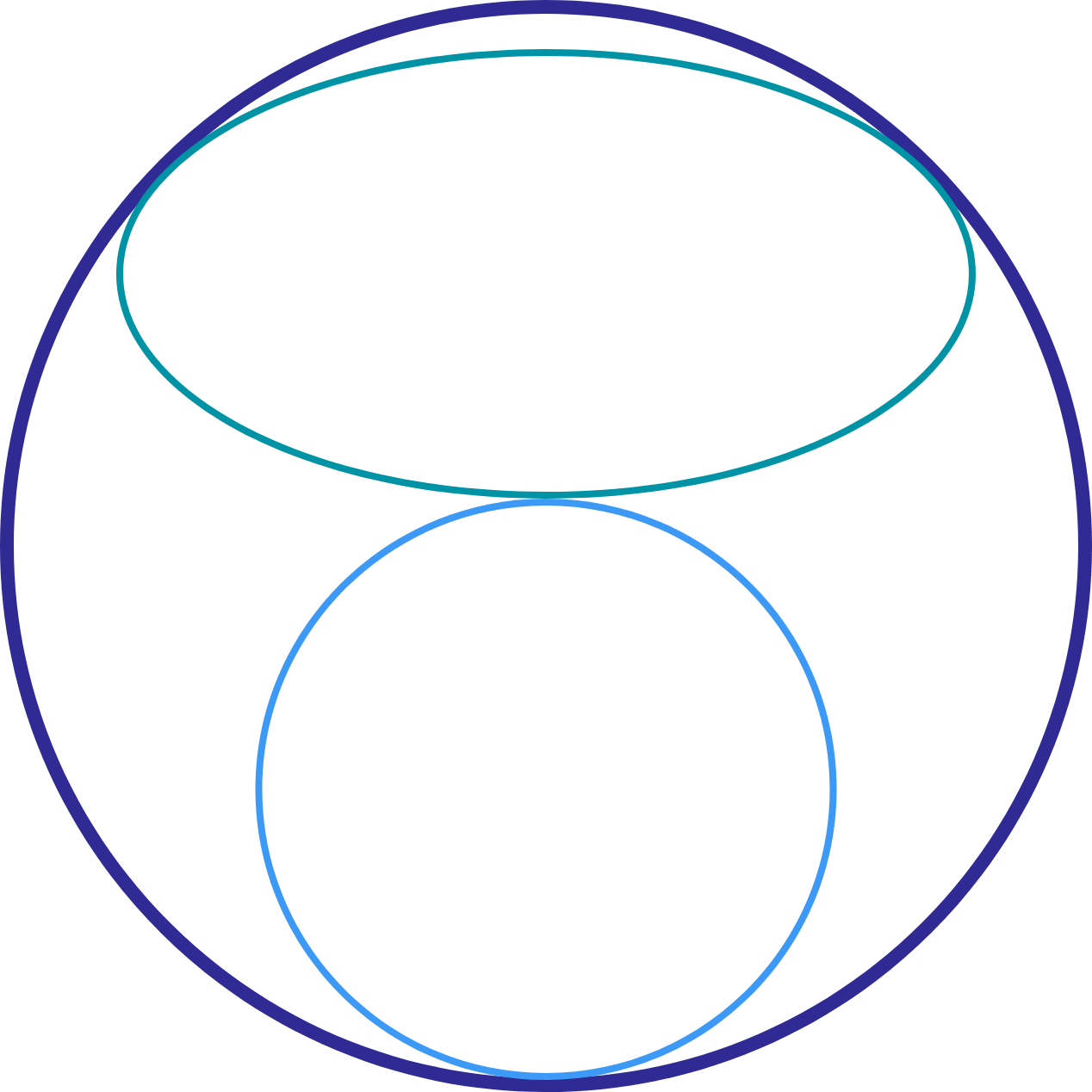For the size of the smaller circle, the ellipse has the largest possible area that could fit in the space between the smaller and larger circle. Let $a$ be the combined areas of the ellipse and the small circle, and let $b$ be the area of the large circle.

Find $\left\lfloor 10000\dfrac { a }{ b } \right\rfloor$

You may want to use a computer for this.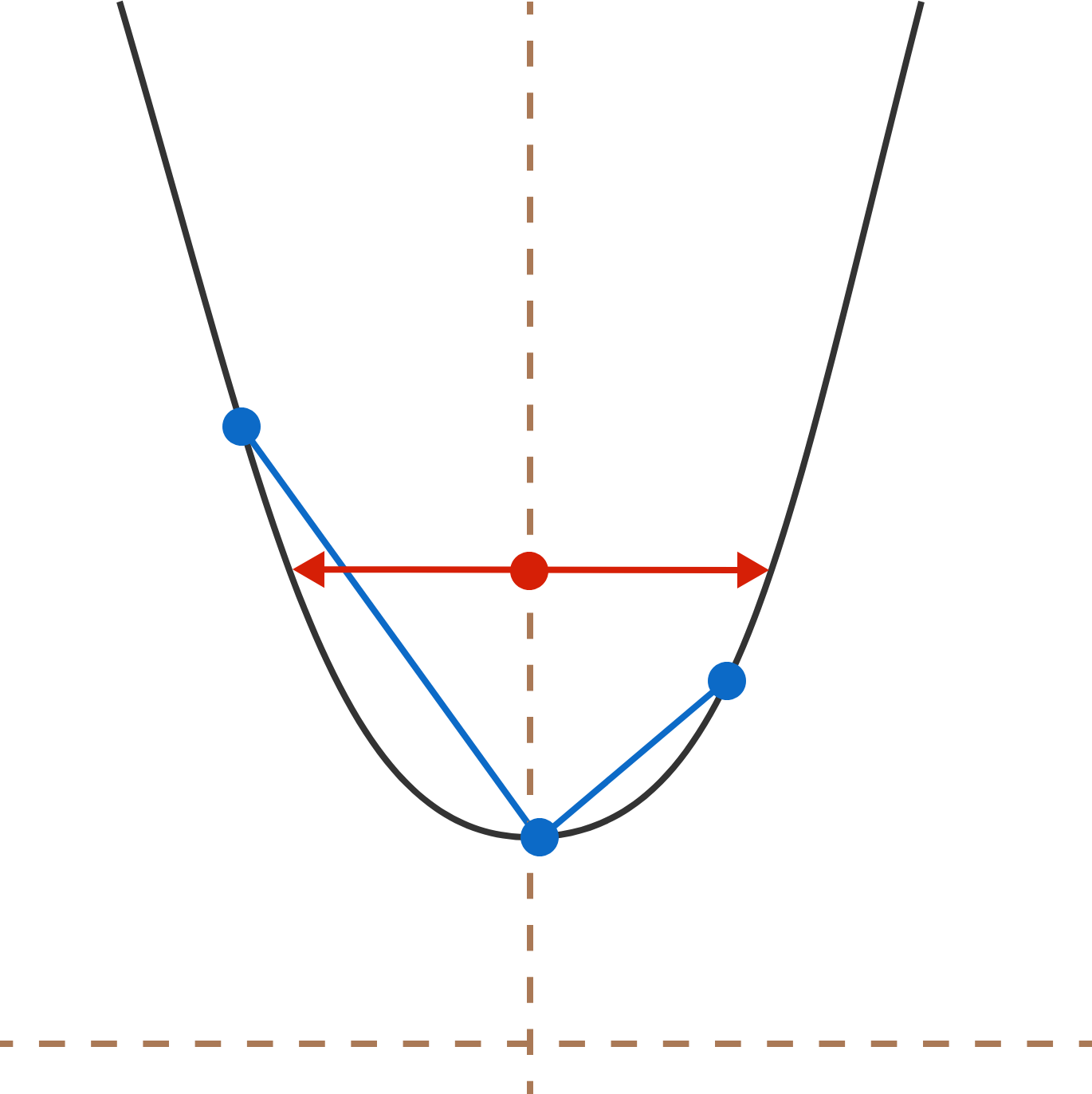Two mutually perpendicular chords are drawn from the vertex of parabola such that their lengths are $8$ and $27$. This is possible for only one distance between the parabola's focus and the directrix.

The length of latus rectum of such a parabola can be expressed as $\displaystyle \frac{a}{\sqrt{b}}$ where $a$ and $b$ are positive coprime integers and $b$ is square-free .

Enter the value of $a+b$.

Details and Assumptions:

• Latus rectum is the focal chord of a parabola which is perpendicular to the axis of the parabola.
×